# Electromotive Force & Internal Resistance

A. Electromotive Force

When a charge passes through the power supply, it gains electrical energy. The power supply is said to have an electromotive force, or emf. Electromotive force is measured in volts. Electromotive force is not a force. Instead, it is the energy gained by the charge that comes from the chemical energy of the battery. In equation form,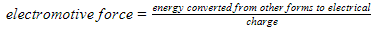B. Internal Resistance

All power supplies have some resistance between their terminals, called internal resistance. Hence, some of the charge circulating in the circuit dissipates some energy in the power supply itself. Because of the current delivered by the power supply, it becomes warm.

Consider Figure 1 given below. The power supply has an emf (E) and internal resistance (r). An external resistor (R) (also called a load) is connected in series. A current (I) flows through the circuit. The voltage across the resistor (R) is VR and the voltage across the internal resistance (r) is Vr.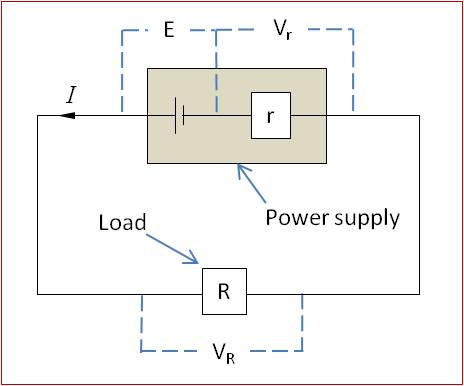Figure 1: Circuit Showing Electromotive Force and Internal Resistance of a Cell

Using the conservation of energy,Hence, the potential across the load, which is the terminal potential difference, isThe terminal potential difference is the potential difference across the terminals of the power supply when a current is delivered.

It can be noted that the terminal potential difference is less than the electromotive force of the power supply when current flows. This is because of the potential lost across the internal resistance. The potential across the internal resistor is called lost volts.The electromotive force is the terminal potential difference when the cell is in an open circuit (no current flows). The emf can be measured by connecting a high-resistance voltmeter across the terminals of the cells, as shown in Figure 2.Figure 2: Measuring Emf of a Cell

This circuit can be used to show that the greater the current delivered by the power supply, the lower its terminal potential difference. As more lamps are connected across the cell, the current increases. The lost volts is given by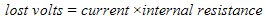The lost voltage also increases with the increase in current. Hence, the terminal potential difference decreases.

According to Figure 1, the emf can be written as: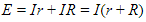From the above equation, it can be noted that the power supply delivers maximum current when it is connected to a load of negligible resistance (R=0). In this case, the terminal potential difference is zero because the potential difference across the internal resistance is equal to the emf of the cell.

C. Effect of Internal Resistance in Power Delivered from a Battery

The power delivered by a battery to a variable load resistor is given by the circuit below.Figure 3: Circuit to Find the Power Delivered to Load

The values of the current and voltage are taken from the ammeter and voltmeter respectively for different values of resistance. The resistance is obtained by the equation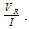. The power is calculated using the equation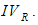The figure below shows the variation of power dissipated with respect to the resistance of the load.

This graph shows that the maximum power is delivered at one value of the load. This value is the internal resistance of the cell.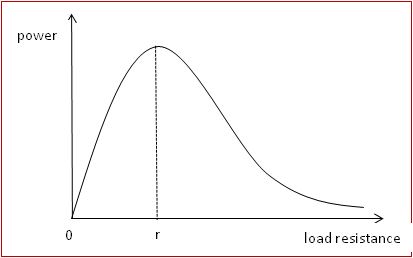Figure 3: Variation of Power Versus Load Resistance

A battery delivers maximum power to a circuit when the load resistance is equal to the internal resistance of the battery.

D. Summary:

• The electromotive force of a supply measures the electrical energy gained per unit of charge passing through the supply.
• The voltage across the terminals of a supply is always less than the emf of the supply when the supply is delivering a current because of the lost volts across the internal resistance.
• For a supply of emf E, which has internal resistance r, E=I(r+R), where R is the external circuit resistance and I is the current in the supply.
• A battery delivers maximum power to a circuit when the load resistance is equal to the internal resistance of the battery.